Counting the Number of Values Below or Above Average

In this article, you will learn how to count the numbers in a range, which are below than average number and above than average number by using Countif function. Along with Average function in Microsoft Excel.

What both functions will do?

Countif function will count the number within range which is less than or above the average.

Average function will provide the criteria (average number of range) to Countif function.

Let’s take an example and understand:-

We have data in Excel, in which we want to count the numbers which are less than Average.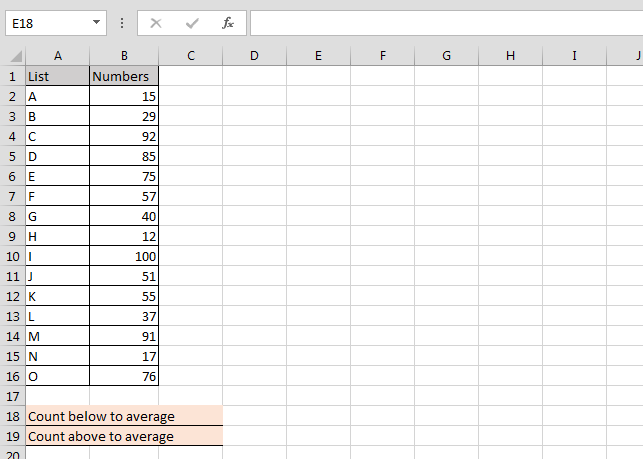Follow below given steps to count the numbers which is below to average:-

• Enter the formula in cell E18
• =COUNTIF(B2:B16,"<"&AVERAGE(B2:B16))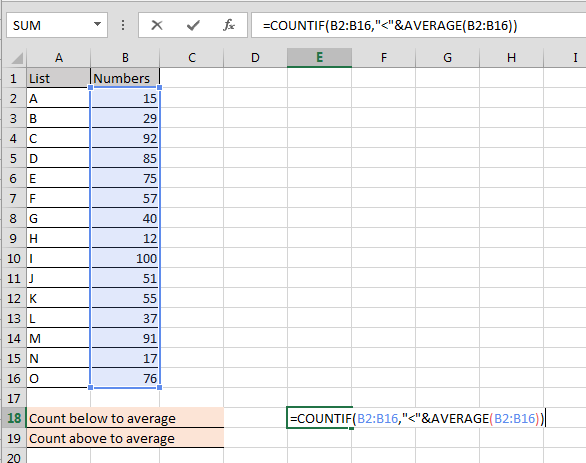• Press Enter
• The function will return the 8 count
• It means within range we have 8 numbers which are below than average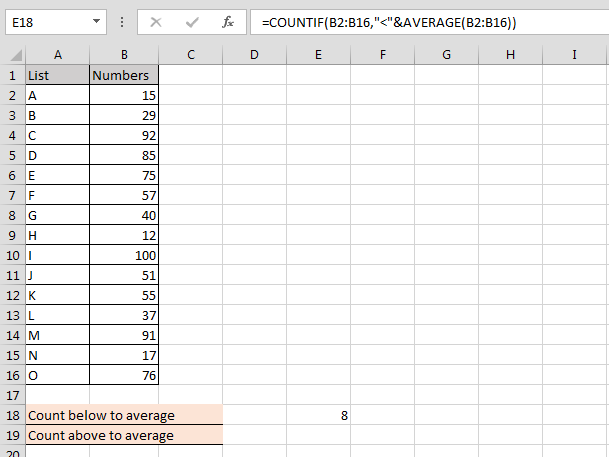Let’s calculate the numbers which are above average:-

• Enter the formula in cell E19
• =COUNTIF(B2:B16,">"&AVERAGE(B2:B16))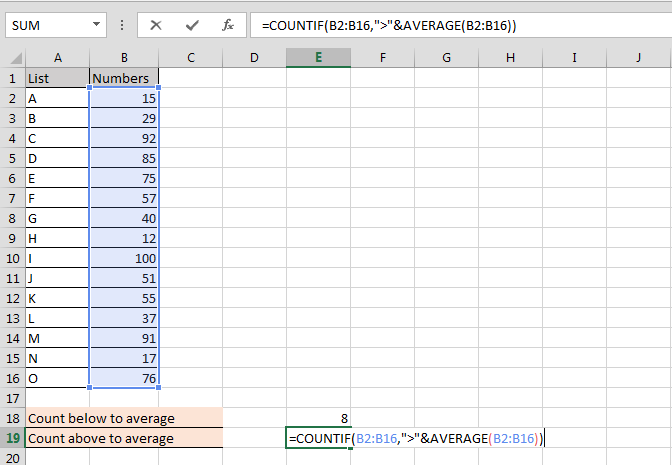• Press Enter
• The function will return 7
• It means, there are 7 numbers which are above average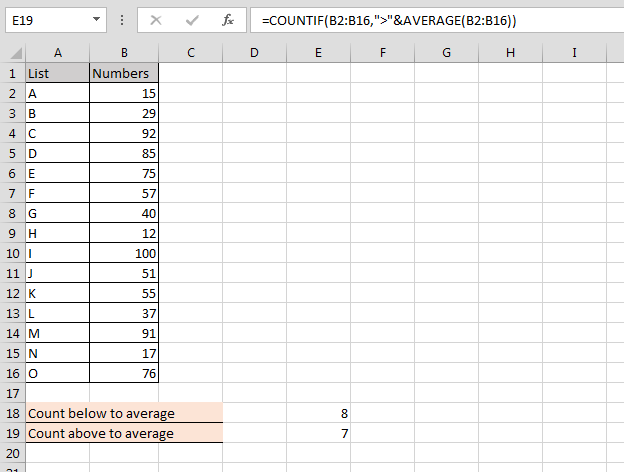We would love to hear from you, do let us know how we can improve, complement or innovate our work and make it better for you. Write us at info@exceltip.com

1.